# 线性回归(回归)

2019-01-10

## 1 概述

### 1.1 批量梯度下降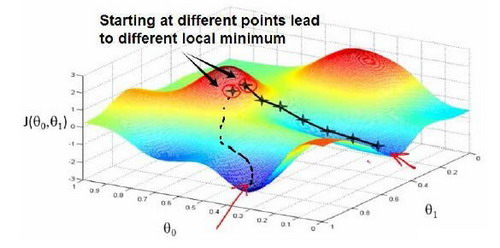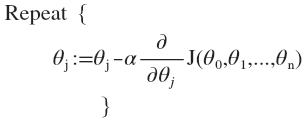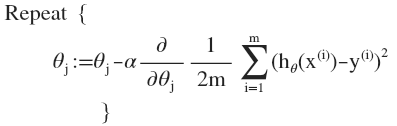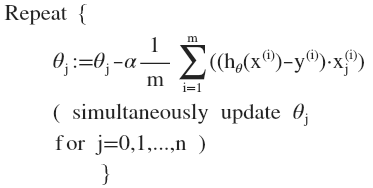$n=1$时，批量梯度下降算法中各参数的计算过程如下：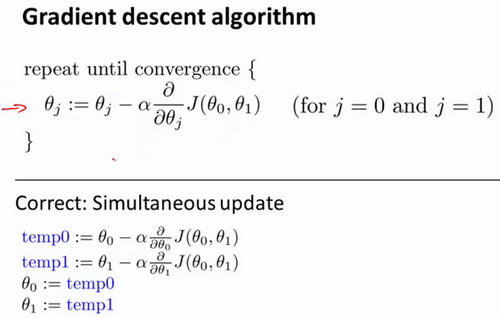• 如果$\alpha$太小了，即我的学习速率太小，$J(\theta_0,\theta_1,...,\theta_n)$的收敛速度很慢，则达到收敛所需的迭代次数会非常高；
• 如果$\alpha$太大了，那么梯度下降法可能会越过最低点，甚至可能无法收敛。

$\theta_n:=\theta_n-\alpha\dfrac{d}{d\theta_n}J(\theta_0,\theta_1,...,\theta_n)$

• 优点：全局最优解；易于并行实现；
• 缺点：当样本数目很多时，训练过程会很慢。

### 1.2 正规方程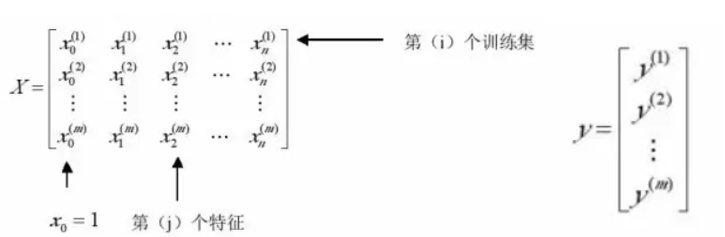1. 特征冗余：如果两个特征$(x_1,x_2)$相关，即可表示为$x_2=f(x_1)$，则会造成$(X^TX)^{-1}$不可逆。
解决办法：剔除掉一个特征。
2. 特征$n$远大于训练集个数$m$:如果训练集10个，而特征$x$有100个$(x_1,x_2,x_3,...,x_{100})$
解决办法：如果可以接受，使用更少的特征建模。

## 2 总结

• 优点：实现简单，计算简单；
• 缺点：不能拟合非线性数据。